# How PROC REPORT Builds a Report

## Sequence of Events

This section explains the general process of building a report. For examples that illustrate this process, see The sequence of events is the same whether you use programming statements or the interactive report window environment.
To understand the process of building a report, you must understand the difference between report variables and temporary variables. Report variables are variables that are specified in the COLUMN statement. A report variable can come from the input data set or can be computed (that is, the DEFINE statement for that variable specifies the COMPUTED option). A report variable might or might not appear in a compute block. Variables that appear only in one or more compute blocks are temporary variables. Temporary variables do not appear in the report and are not written to the output data set (if one is requested).
PROC REPORT constructs a report as follows:
1. It consolidates the data by group, order, and across variables. It calculates all statistics for the report, the statistics for detail rows as well as the statistics for summary lines in breaks. Statistics include those statistics that are computed for analysis variables. PROC REPORT calculates statistics for summary lines whether they appear in the report.
2. It initializes all temporary variables to missing.
3. It begins constructing the rows of the report.
1. At the beginning of each row, it initializes all report variables to missing.
2. It fills in values for report variables from left to right.
• Values for computed variables come from executing the statements in the corresponding compute blocks.
• Values for all other variables come from the data set or the summary statistics that were computed at the beginning of the report-building process.
3. Whenever it comes to a break, PROC REPORT first constructs the break lines that are created with the BREAK or RBREAK statement or with options in the BREAK window. If there is a compute block attached to the break, then PROC REPORT then executes the statements in the compute block. See for details.
Note: Because of the way PROC REPORT builds a report, you can
• use group statistics in compute blocks for a break before the group variable.
• use statistics for the whole report in a compute block at the beginning of the report.
This document references these statistics with the appropriate compound name. For information about referencing report items in a compute block, see
Note: You cannot use the LINE statement in conditional statements (IF-THEN, IF-THEN/ELSE, and SELECT) because it is not executed until PROC REPORT has executed all other statements in the compute block.
4. After each report row is completed, PROC REPORT sends the row to all of the ODS destinations that are currently open.

## Construction of Summary Lines

PROC REPORT constructs a summary line for a break if either of the following conditions is true:
• You summarize numeric variables in the break.
• You use a compute block at the break. (You can attach a compute block to a break without using a BREAK or RBREAK statement or without selecting any options in the BREAK window.)
The summary line that PROC REPORT constructs at this point is preliminary. If no compute block is attached to the break, then the preliminary summary line becomes the final summary line. However, if a compute block is attached to the break, then the statements in the compute block can alter the values in the preliminary summary line.
PROC REPORT prints the summary line only if you summarize numeric variables in the break.

## Report-Building Examples

### Building a Report That Uses Groups and a Report Summary

The report in contains five columns:
• Sector and Department are group variables.
• Sales is an analysis variable that is used to calculate the Sum statistic.
• Profit is a computed variable whose value is based on the value of Department.
• The N statistic indicates how many observations each row represents.
At the end of the report a break summarizes the statistics and computed variables in the report and assigns to Sector the value of `TOTALS:`.
The following statements produce The user-defined formats that are used are created by a
```libname proclib
'SAS-library';```
```options nodate pageno=1 linesize=64
pagesize=60 fmtsearch=(proclib);

ods html close;
ods listing;
column sector department sales Profit N ;
define sector / group format=\$sctrfmt.;
define department   / group format=\$deptfmt.;
define sales  / analysis sum
format=dollar9.2;
define profit / computed format=dollar9.2;

compute before;
totprof = 0;
endcomp;

compute profit;
if sector ne ' ' or department ne ' ' then do;
if department='np1' or department='np2'
then profit=0.4*sales.sum;
else profit=0.25*sales.sum;
totprof = totprof + profit;
end;
else
profit = totprof;
endcomp;

rbreak after / dol dul summarize;
compute after;
sector='TOTALS:';
endcomp;

where sector contains 'n';
title 'Report for Northeast and Northwest Sectors';
run;
ods listing close;```
Report with Groups and a Report Summary
```
Report for Northeast and Northwest Sectors          1

Sector     Department      Sales     Profit          N
------------------------------------------------------

Northeast  Canned        \$840.00    \$336.00          2
Meat/Dairy    \$490.00    \$122.50          2
Paper         \$290.00     \$116.00         2
Produce       \$211.00     \$52.75          2
Northwest  Canned      \$1,070.00    \$428.00          3
Meat/Dairy  \$1,055.00    \$263.75          3
Paper         \$150.00     \$60.00          3
Produce       \$179.00     \$44.75          3
=========              =========  =========  =========
TOTALS:                \$4,285.00  \$1,423.75         20
=========              =========  =========  =========
```
A description of how PROC REPORT builds this report follows:
1. PROC REPORT starts building the report by consolidating the data (Sector and Department are group variables) and by calculating the statistics (Sales.sum and N) for each detail row and for the break at the end of the report.
2. Now, PROC REPORT is ready to start building the first row of the report. This report does not contain a break at the beginning of the report or a break before any groups, so the first row of the report is a detail row. The procedure initializes all report variables to missing, as the following figure illustrates. Missing values for a character variable are represented by a blank, and missing values for a numeric variable are represented by a period.
First Detail Row with Values Initialized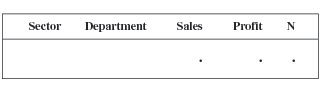3. The following figure illustrates the construction of the first three columns of the row. PROC REPORT fills in values for the row from left to right. Values come from the statistics that were computed at the beginning of the report-building process.
First Detail Row with Values Filled in from Left to Right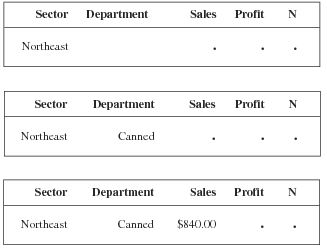4. The next column in the report contains the computed variable Profit. When it gets to this column, PROC REPORT executes the statements in the compute block that is attached to Profit. Nonperishable items (which have a value of `np1` or `np2`) return a profit of 40%; perishable items (which have a value of `p1` or `p2`) return a profit of 25%.
```   if department='np1' or department='np2'
then profit=0.4*sales.sum;
else profit=0.25*sales.sum;```
The row now looks like the following figure.
Note: The position of a computed variable is important. PROC REPORT assigns values to the columns in a row of a report from left to right. Consequently, you cannot base the calculation of a computed variable on any variable that appears to its right in the report.
A Computed Variable Added to the First Detail Row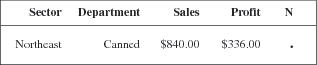5. Next, PROC REPORT fills in the value for the N statistic. The value comes from the statistics that are created at the beginning of the report-building process. The following figure illustrates the completed row.
First Complete Detail Row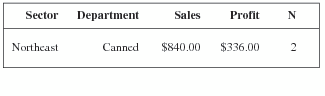6. The procedure writes the completed row to the report.
7. PROC REPORT repeats steps 2, 3, 4, 5, and 6 for each detail row in the report.
8. At the break at the end of the report, PROC REPORT constructs the break lines described by the RBREAK statement. These lines include double underlining, double overlining, and a preliminary version of the summary line. The statistics for the summary line were calculated earlier. (See step 1.) The value for the computed variable is calculated when PROC REPORT reaches the appropriate column, just as it is in detail rows. PROC REPORT uses these values to create the preliminary version of the summary line. (See the following figure.)
Preliminary Summary Line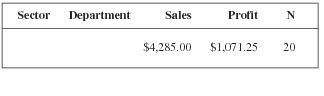9. If no compute block is attached to the break, then the preliminary version of the summary line is the same as the final version. However, in this example, a compute block is attached to the break. Therefore, PROC REPORT now executes the statements in that compute block. In this case, the compute block contains one statement:
`   sector='TOTALS:';`
This statement replaces the value of Sector, which in the summary line is missing by default, with the word `TOTALS:`. After PROC REPORT executes the statement, it modifies the summary line to reflect this change to the value of Sector. The final version of the summary line appears in the following figure.
Final Summary Line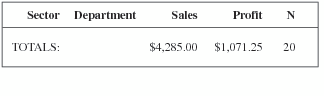10. Finally, PROC REPORT writes all the break lines, with underlining, overlining, and the final summary line, to the report.

### Building a Report That Uses Temporary Variables

PROC REPORT initializes report variables to missing at the beginning of each row of the report. The value for a temporary variable is initialized to missing before PROC REPORT begins to construct the rows of the report, and it remains missing until you specifically assign a value to it. PROC REPORT retains the value of a temporary variable from the execution of one compute block to another.
Because all compute blocks share the current values of all variables, you can initialize temporary variables at a break at the beginning of the report or at a break before a break variable. This report initializes the temporary variable Sctrtot at a break before Sector.
Note: PROC REPORT creates a preliminary summary line for a break before it executes the corresponding compute block. If the summary line contains computed variables, then the computations are based on the values of the contributing variables in the preliminary summary line. If you want to recalculate computed variables based on values that you set in the compute block, then you must do so explicitly in the compute block. This report illustrates this technique. If no compute block is attached to a break, then the preliminary summary line becomes the final summary line.
The report in contains five columns:
• Sector and Department are group variables.
• Sales is an analysis variable that is used twice in this report: once to calculate the Sum statistic, and once to calculate the Pctsum statistic.
• Sctrpct is a computed variable whose values are based on the values of Sales and a temporary variable, Sctrtot, which is the total sales for a sector.
At the beginning of the report, a customized report summary tells what the sales for all stores are. At a break before each group of observations for a department, a default summary summarizes the data for that sector. At the end of each group a break inserts a blank line.
The following statements produce The user-defined formats that are used are created by a
Note: Calculations of the percentages do not multiply their results by 100 because PROC REPORT prints them with the PERCENT. format.
```libname proclib
'SAS-library';```
```options nodate pageno=1 linesize=64
pagesize=60 fmtsearch=(proclib);
ods html close;
ods listing;
column sector department sales
Sctrpct sales=Salespct;

define sector     / 'Sector' group
format=\$sctrfmt.;
define department / group format=\$deptfmt.;
define sales      / analysis sum
format=dollar9.2 ;
define sctrpct    / computed
format=percent9.2 ;
define salespct   / pctsum format=percent9.2;

compute before;
line ' ';
line @16 'Total for all stores is '
sales.sum dollar9.2;
line ' ';
line @29 'Sum of' @40 'Percent'
@51 'Percent of';
line @6 'Sector' @17 'Department'
@29 'Sales'
@40 'of Sector' @51 'All Stores';
line @6 55*'=';
line ' ';
endcomp;

break before sector / summarize ul;
compute before sector;
sctrtot=sales.sum;
sctrpct=sales.sum/sctrtot;
endcomp;

compute sctrpct;
sctrpct=sales.sum/sctrtot;
endcomp;

break after sector/skip;
where sector contains 'n';
title 'Report for Northeast and Northwest Sectors';
run;
ods listing close;```
Report with Temporary Variables
```
Report for Northeast and Northwest Sectors          1

Total for all stores is \$4,285.00

Sum of     Percent    Percent of
Sector     Department  Sales      of Sector  All Stores
=======================================================

Northeast              \$1,831.00   100.00%     42.73%
---------              ---------  ---------  ---------
Northeast  Canned        \$840.00    45.88%     19.60%
Meat/Dairy    \$490.00    26.76%     11.44%
Paper         \$290.00    15.84%      6.77%
Produce       \$211.00    11.52%      4.92%

Northwest              \$2,454.00   100.00%     57.27%
---------              ---------  ---------  ---------
Northwest  Canned      \$1,070.00    43.60%     24.97%
Meat/Dairy  \$1,055.00    42.99%     24.62%
Paper         \$150.00     6.11%      3.50%
Produce       \$179.00     7.29%      4.18%

```
A description of how PROC REPORT builds this report follows:
1. PROC REPORT starts building the report by consolidating the data (Sector and Department are group variables) and by calculating the statistics (Sales.sum and Sales.pctsum) for each detail row, for the break at the beginning of the report, for the breaks before each group, and for the breaks after each group.
2. PROC REPORT initializes the temporary variable, Sctrtot, to missing. (See the following figure.)
Initialized Temporary Variables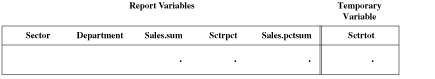3. Because this PROC REPORT step contains a COMPUTE BEFORE statement, the procedure constructs a preliminary summary line for the break at the beginning of the report. This preliminary summary line contains values for the statistics (Sales.sum and Sales.pctsum) and the computed variable (Sctrpct).
At this break, Sales.sum is the sales for all stores, and Sales.pctsum is the percentage those sales represent for all stores (100%). PROC REPORT takes the values for these statistics from the statistics that were computed at the beginning of the report-building process.
The value for Sctrpct comes from executing the statements in the corresponding compute block. Because the value of Sctrtot is missing, PROC REPORT cannot calculate a value for Sctrpct. Therefore, in the preliminary summary line (which is not printed in this case), this variable also has a missing value. (See the following figure.)
The statements in the COMPUTE BEFORE block do not alter any variables. Therefore, the final summary line is the same as the preliminary summary line.
Note: The COMPUTE BEFORE statement creates a break at the beginning of the report. You do not need to use an RBREAK statement.
Preliminary and Final Summary Line for the Break at the Beginning of the Report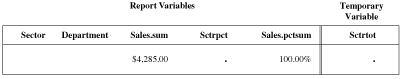4. Because the program does not include an RBREAK statement with the SUMMARIZE option, PROC REPORT does not write the final summary line to the report. Instead, it uses LINE statements to write a customized summary that embeds the value of Sales.sum into a sentence and to write customized column headings. (The NOHEADER option in the PROC REPORT statement suppresses the default column headings, which would have appeared before the customized summary.)
5. Next, PROC REPORT constructs a preliminary summary line for the break before the first group of observations. (This break both uses the SUMMARIZE option in the BREAK statement and has a compute block attached to it. Either of these conditions generates a summary line.) The preliminary summary line contains values for the break variable (Sector), the statistics (Sales.sum and Sales.pctsum), and the computed variable (Sctrpct). At this break, Sales.sum is the sales for one sector (the northeast sector). PROC REPORT takes the values for Sector, Sales.sum, and Sales.pctsum from the statistics that were computed at the beginning of the report-building process.
The value for Sctrpct comes from executing the statements in the corresponding compute blocks. Because the value of Sctrtot is still missing, PROC REPORT cannot calculate a value for Sctrpct. Therefore, in the preliminary summary line, Sctrpct has a missing value. (See the following figure.)
Preliminary Summary Line for the Break before the First Group of Observations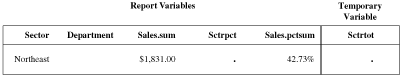6. PROC REPORT creates the final version of the summary line by executing the statements in the COMPUTE BEFORE SECTOR compute block. These statements execute once each time the value of Sector changes.
• The first statement assigns the value of Sales.sum, which in that part of the report represents total sales for one Sector, to the variable Sctrtot.
• The second statement completes the summary line by recalculating Sctrpct from the new value of Sctrtot. The following figure shows the final summary line.
Note: In this example, you must recalculate the value for Sctrpct in the final summary line. If you do not recalculate the value for Sctrpct, then it will be missing because the value of Sctrtot is missing at the time that the COMPUTE Sctrpct block executes.
Final Summary Line for the Break before the First Group of Observations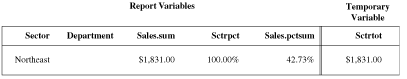7. Because the program contains a BREAK BEFORE statement with the SUMMARIZE option, PROC REPORT writes the final summary line to the report. The UL option in the BREAK statement underlines the summary line.
8. Now, PROC REPORT is ready to start building the first report row. It initializes all report variables to missing. Values for temporary variables do not change. The following figure illustrates the first detail row at this point.
First Detail Row with Initialized Values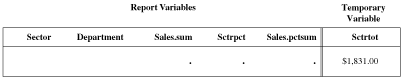9. The following figure illustrates the construction of the first three columns of the row. PROC REPORT fills in values for the row from left to right. The values come from the statistics that were computed at the beginning of the report-building process.
Filling in Values from Left to Right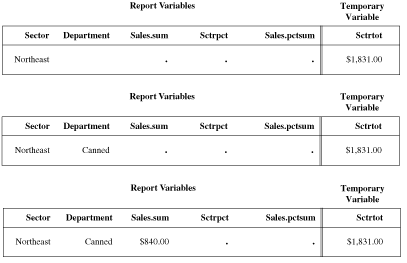10. The next column in the report contains the computed variable Sctrpct. When it gets to this column, PROC REPORT executes the statement in the compute block attached to Sctrpct. This statement calculates the percentage of the sector's total sales that this department accounts for:
`   sctrpct=sales.sum/sctrtot;`
The row now looks like the following figure.
First Detail Row with the First Computed Variable Added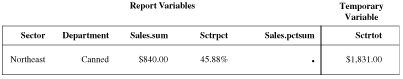11. The next column in the report contains the statistic Sales.pctsum. PROC REPORT gets this value from the statistics that are created at the beginning of the report-building process. The first detail row is now complete. (See the following figure.)
First Complete Detail Row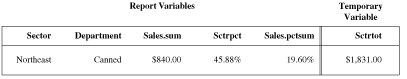12. PROC REPORT writes the detail row to the report. It repeats steps 8, 9, 10, 11, and 12 for each detail row in the group.
13. After writing the last detail row in the group to the report, PROC REPORT constructs the default group summary. Because no compute block is attached to this break and because the BREAK AFTER statement does not include the SUMMARIZE option, PROC REPORT does not construct a summary line. The only action at this break is that the SKIP option in the BREAK AFTER statement writes a blank line after the last detail row of the group.
14. Now the value of the break variable changes from `Northeast` to `Northwest`. PROC REPORT constructs a preliminary summary line for the break before this group of observations. As at the beginning of any row, PROC REPORT initializes all report variables to missing but retains the value of the temporary variable. Next, it completes the preliminary summary line with the appropriate values for the break variable (Sector), the statistics (Sales.sum and Sales.pctsum), and the computed variable (Sctrpct). At this break, Sales.sum is the sales for the Northwest sector. Because the COMPUTE BEFORE Sector block has not yet executed, the value of Sctrtot is still \$1,831.00, the value for the Northeast sector. Thus, the value that PROC REPORT calculates for Sctrpct in this preliminary summary line is incorrect. (See the following figure.) The statements in the compute block for this break calculate the correct value. (See the following step.)
Preliminary Summary Line for the Break before the Second Group of Observations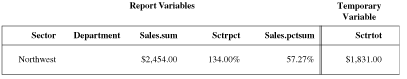CAUTION:
Synchronize values for computed variables in break lines to prevent incorrect results.
If the PROC REPORT step does not recalculate Sctrpct in the compute block that is attached to the break, then the value in the final summary line will not be synchronized with the other values in the summary line, and the report will be incorrect.
15. PROC REPORT creates the final version of the summary line by executing the statements in the COMPUTE BEFORE Sector compute block. These statements execute once each time the value of Sector changes.
• The first statement assigns the value of Sales.sum, which in that part of the report represents sales for the Northwest sector, to the variable Sctrtot.
• The second statement completes the summary line by recalculating Sctrpct from the new, appropriate value of Sctrtot. The following figure shows the final summary line.
Final Summary Line for the Break before the Second Group of Observations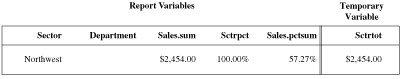Because the program contains a BREAK BEFORE statement with the SUMMARIZE option, PROC REPORT writes the final summary line to the report. The UL option in the BREAK statement underlines the summary line.
16. Now, PROC REPORT is ready to start building the first row for this group of observations. It repeats steps 8 through 16 until it has processed all observations in the input data set (stopping with step 14 for the last group of observations).